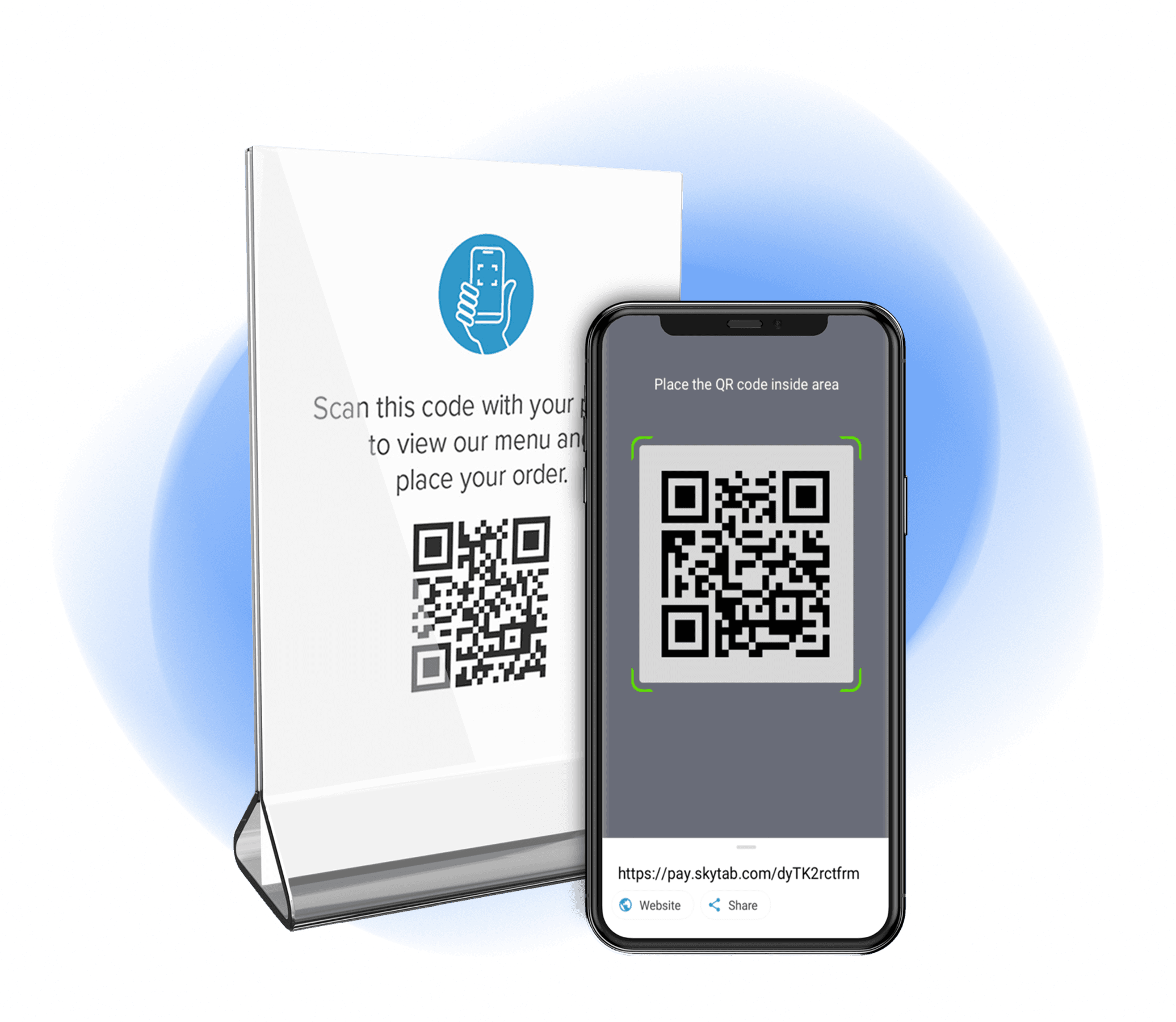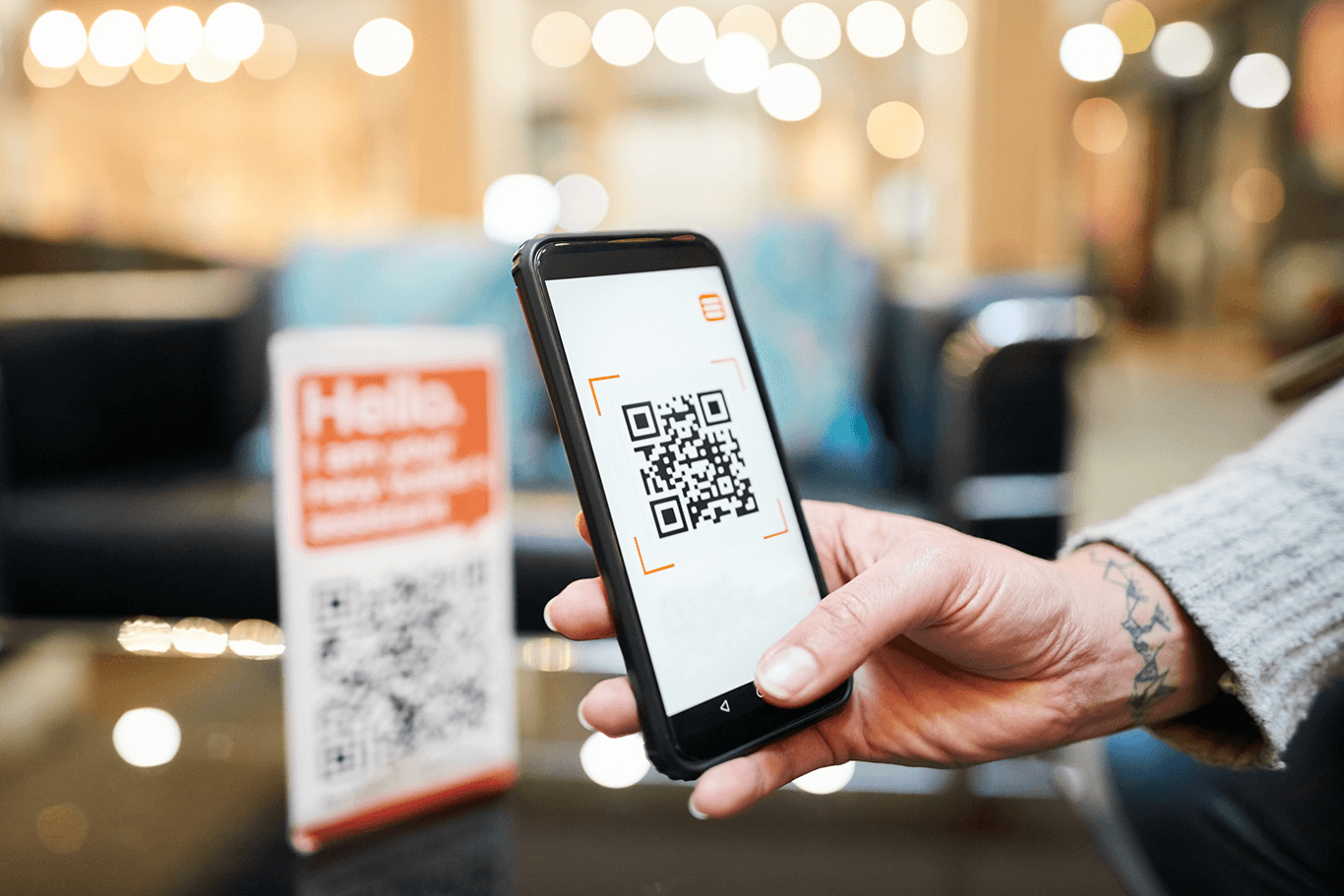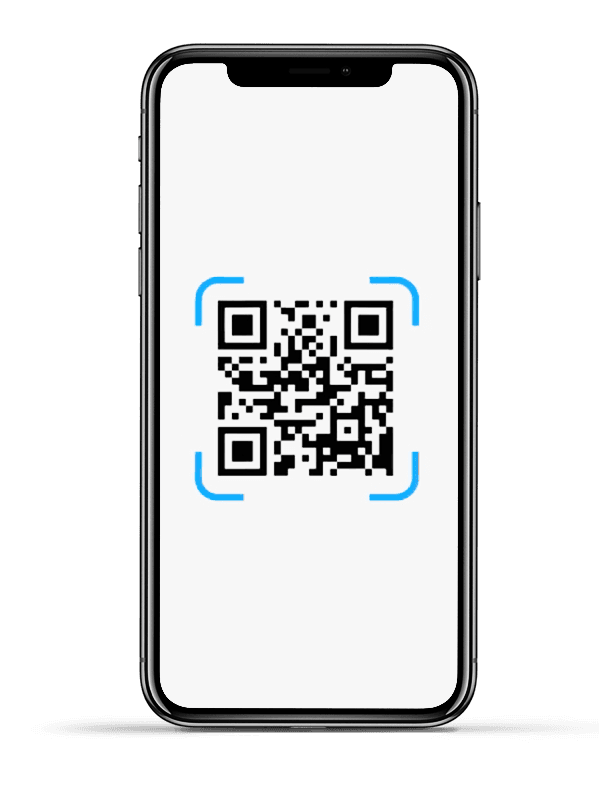# QR Codes Deliver Contactless Convenienceself.__wrap_balancer=(t,e,n)=>{n=n||document.querySelector(`[data-br="\${t}"]`);let o=n.parentElement,r=E=>n.style.maxWidth=E+"px";n.style.maxWidth="";let i=o.clientWidth,s=o.clientHeight,c=i/2,u=i,d;if(i){for(;c+1<u;)d=~~((c+u)/2),r(d),o.clientHeight==s?u=d:c=d;r(u*e+i*(1-e))}};self.__wrap_balancer(":R2hlm6:",1)

Take orders and accept payments at the table with QR code technology.# Order With QR Codesself.__wrap_balancer=(t,e,n)=>{n=n||document.querySelector(`[data-br="\${t}"]`);let o=n.parentElement,r=E=>n.style.maxWidth=E+"px";n.style.maxWidth="";let i=o.clientWidth,s=o.clientHeight,c=i/2,u=i,d;if(i){for(;c+1<u;)d=~~((c+u)/2),r(d),o.clientHeight==s?u=d:c=d;r(u*e+i*(1-e))}};self.__wrap_balancer(":R2ilm6:",1)

Serve up a contactless ordering experience.

## Ready to Order? Just scan.self.__wrap_balancer=(t,e,n)=>{n=n||document.querySelector(`[data-br="\${t}"]`);let o=n.parentElement,r=E=>n.style.maxWidth=E+"px";n.style.maxWidth="";let i=o.clientWidth,s=o.clientHeight,c=i/2,u=i,d;if(i){for(;c+1<u;)d=~~((c+u)/2),r(d),o.clientHeight==s?u=d:c=d;r(u*e+i*(1-e))}};self.__wrap_balancer(":Rb3lm6:",1)

• Display a unique SkyTab-provided QR code at the table
• Customers scan the code with their phone to view the menu
• Customers select their menu items and submit the order
• Orders sync directly to your SkyTab POS system# Pay With QR Codesself.__wrap_balancer=(t,e,n)=>{n=n||document.querySelector(`[data-br="\${t}"]`);let o=n.parentElement,r=E=>n.style.maxWidth=E+"px";n.style.maxWidth="";let i=o.clientWidth,s=o.clientHeight,c=i/2,u=i,d;if(i){for(;c+1<u;)d=~~((c+u)/2),r(d),o.clientHeight==s?u=d:c=d;r(u*e+i*(1-e))}};self.__wrap_balancer(":R2klm6:",1)

Dish out a contactless payment experience.

## Ready to Pay? Just scan.self.__wrap_balancer=(t,e,n)=>{n=n||document.querySelector(`[data-br="\${t}"]`);let o=n.parentElement,r=E=>n.style.maxWidth=E+"px";n.style.maxWidth="";let i=o.clientWidth,s=o.clientHeight,c=i/2,u=i,d;if(i){for(;c+1<u;)d=~~((c+u)/2),r(d),o.clientHeight==s?u=d:c=d;r(u*e+i*(1-e))}};self.__wrap_balancer(":Rb5lm6:",1)

• QR code prints on the check or display it on SkyTab Mobile
• Customers scan the code with their phone to view the bill
• Customers pay with Apple Pay, Google Pay, or their credit card
• The check automatically closes in the POS systemStreamline operations and deliver a better customer experience with QR code technology that integrates directly with SkyTab.

### INCREASE EFFICIENCY

Reduce labor costs and do more with less – and improve the guest experience in the process.